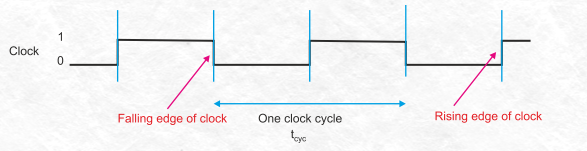$\require{cancel}$

# Architecture Computations

• Generally we are interested in questions involving computer speed.
• What are the units of speed in a computer?
• We will look at different ways you could possibly build a computer.
• We will want to be able to discuss performance of different options so we can compare.
• To do this, we must define what performance is.
• We will discuss performance in some detail later, but for now we will define it to be time spent on a program.
• The basic components of time in a program.
• The clock
• The number of instructions in the program.
• The number of cycles per instruction (CPI)
• The clock
• Discussed on page 33 of the book.
• Discussed in appendix B.7
• This is the driver of the CPU
• It is an electronic signal that changes between a low signal and a high signal.
• This could be a sin/cos wave, or probably a shaped.
• A clock cycle
• Is one iteration of the clock.
• It generally has a rising edge, where most logic takes place.
• But we can shape the clock if we wish, more on that later.
•(From alanclements.org)
• The clock frequency is the amount of cycles run in a given time period.
• Normally this is cycles per second.
• The unit for this is Hertz (Hz).
• A 1 Hz Clock has a frequency of one cycle per second.
• $\frac{\text{cycles}}{\text{second}}$
• What are typical clock rates?
• Clock cycle time
• This is $\frac{\text{seconds}}{\text{cycle}}$
• My windows machine claims to run at 2.6GHz, how long is each cycle?
• $2.6GHz = \frac{2.6\times10^{9}\text{cycles}}{1\text{second}}$.
• That is the same as $\frac{1\text{second}}{2.6\times10^{9}\text{cycles}}$
• Or $\frac{1}{2.6} \times 10^{-9} \frac{\text{seconds}}{\text{cycle}}$
• or $0.38 \times 10^{-9} \frac{\text{seconds}}{\text{cycle}}$
• So a cycle is $0.38 \times 10^{-9} \text{seconds}$.
• So a cycle is $3.8 \times 10^{-10} \text{seconds}$.
• This doesn't seem like a good answer, let's change units.
• What unit do I want? (nano seconds or pico seconds?) (pico)
• $1 \text{second} = 1 \times 10^{12} \text{pico seconds}$
• $3.8 \times 10^{-10} \text{seconds} \times \frac{1 \times 10^{12} \text{pico seconds}}{1 \text{seconds}}$
• $3.8 \times 10^{\cancel{-10} 1} \cancel{\text{seconds}} \times \frac{1 \times 10^{\cancel{12} 2} \text{pico seconds}}{1 \cancel{\text{seconds}}}$ That 12 should be canceled
• Or $3.8 \times 10^2$ps
• Or 380 ps/cycle.
• Why is this important?
• this is, in theory, how long it will take to execute a single instruction!
• The number of instructions per program.
• These are machine/assembly instructions.
• We will be able to tell the difference later, but for now you can think of them as semi-equivalent.
• Right now we will consider all instructions of equal weight.
• We will discover that this is not the case later.
• We will discuss this in more detail later.
• The final part of the mix is CPI or IPC .
• Cycles per instruction.
• Ideally we would like to be able to execute an instruction every cycle.
• But we might not be able to do this.